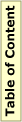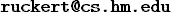## MMIX Bug Report Rounding of floating point numbersmmix-20131017

### Bug Reported

Initial: 17/7/2015

Joe Zbiciak

### Description

In special cases the code in section 49 of mmix-arith marked as "tricky but OK" will perform incorrect rounding. For Example the following code produces this error:
```        LOC     Data_Segment
GREG    @
PassMsg BYTE    "PASS",10,0
FailMsg BYTE    "FAIL",10,0

LOC     #100

Main    PUT     rA,#0     % rA = 0 establishes round-to-even.
SETH    \$2,#C000  % \$2 = -1.0 x 2^1
SETH    \$1,#3CA8  % \$1 = +1.5 x 2^-53
FADD    \$0,\$1,\$2  % \$0 = -1.0 x 2^1 + +1.5 x 2^-53

SUB     \$3,\$2,\$0  % \$0 should be 1 ulp less than \$2
SUB     \$4,\$3,1   % \$4 should be 0 on success.
BZ      \$4,Pass

Fail    LDA     \$255,FailMsg
TRAP    0,Fputs,StdOut
TRAP    0,Halt,0

Pass    LDA     \$255,PassMsg
TRAP    0,Fputs,StdOut
TRAP    0,Halt,0
```
Here's another example of a failing test:

80F0000000000000 + 0000000000001716 = 80EFFFFFFFFFFFFF vs. 80F0000000000000

In this test, the exponent of the two numbers differs by precisely 54.

The problem is in the line marked with "tricky but OK" in section 49, "Adjust for differences in exponents" of mmix-arith:

```{
if (d <= 2) zf = shift_right (zf , d, 1); /* exact result */
else if (d > 53) zf.h = 0, zf.l = 1; /* tricky but OK */
else {
if (ys != zs) d−−, xe−−, yf = shift_left (yf, 1);
o = zf ;
zf = shift_right (o, d, 1);
oo = shift_left (zf, d);
if (oo.l != o.l || oo.h != o.h) zf.l |= 1;
}
}
```
For this input, yf = 2^54, zf > 2^54 and d = 54. The highlighted case replaces zf with 1, the "sticky bit."

But, because the signs differ (ys != zs), we need a guard bit to do the subtract correctly.

What the current code does is subtract 1 from 2^54, and then round that back up to 2^54.

If we had not gone through the "tricky but OK" condition, and instead proceeded to the final else case, yf would have been promoted to 2^55, and zf would become 3. That result looks like 0x7FFFFFFFFFFFFD, which will round down as the two LSBs are 01.

In the existing code, we have:

```  else if (d > 53) zf.h = 0, zf.l = 1; /* tricky but OK */
```
This actually works correctly when zs == ys. When d == 54 and zs == ys, the code behaves correctly. The misbehavior begins when d == 54 and ys != zs. That's the case that benefits from the extra guard bit obtained by shifting yf left by 1 bit in the general case.

### Proposed Patch

In
```  else if (d > 53) zf.h = 0, zf.l = 1; /* tricky but OK */
```
change the "53" to "54", that seems to correct the issue. That line is mostly an optimization anyway.

### Discussion

For a more exact condition, one could write:
```  else if (d > 54 || ( ys == zs && d > 53 ) ) zf.h = 0, zf.l = 1; /* tricky but OK */
```
Because most of the time we have d<=53, it is more efficient to write
```  else if (d > 53 && ( ys == zs || d > 54 ) ) zf.h = 0, zf.l = 1; /* tricky but OK */
```
The simplest solution: replace d > 53 by d > 54

### Status

Fixed in the repository, Rev. 94, on 2015-08-05.

Please help to keep this site up to date! If you want to point out important material or projects that are not listed here, if you find errors or want to suggest improvements, please send email to